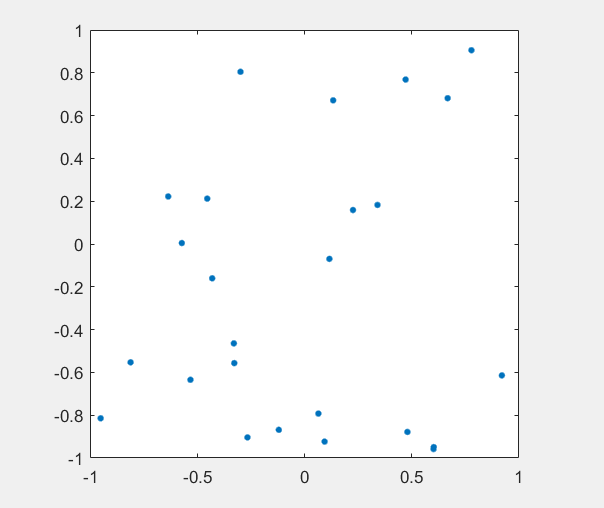# MATLAB 中gcf、gca 以及gco 的区别

+关注继续查看

1.三者的功能定义：

 1 2 3 gcf 返回当前Figure 对象的句柄值 gca 返回当前axes 对象的句柄值 gco 返回当前鼠标单击的句柄值，该对象可以是除root 对象外的任意图形对象，并且Matlab 会把当前图形对象的句柄值存放在Figure 的CurrentObject属性中。

2.matlab下的图形对象

 对象 描述 Root 对计算机屏幕最高级的对象 Figure 用来显示图形和用户界面的窗口 Axes 在窗口中显示图形的轴 Uicontrol 用户界面控制。执行一个对用户交互作用的函数。 Uimenu 用户定义窗口菜单 Uicontextmenu 右键单击对象时弹出的菜单 Image 二维像素基础图 Light 影响斑点和表面对象着色的光源 Line 用于诸如plot, plot3, semilogx 等函数的线 Patch 带边缘的填充多边形 Rectangle 二维形状，从矩形到椭圆 Surface 由x-y 平面以上的数据值所作图创建的矩阵数据的三维表示 Text 字符串

3. 图形对象级别

MATLAB 用户指南给人的一种印象是，句柄图形非常复杂，只对熟练的高级用户才有用。而实际上不是这样的。句柄图形可以被任何人用来改变MATLAB生成图形的方式，不论是只想在一幅图里做一点小变动，还是想做影响所有图形输出的全局变动。

在这个背景下，在本章最后给出了关于句柄图形对象属性和它们的值，它不仅很有用也很有意义。

4. 谁需要句柄图形？

现在，对于那些还在犹豫的用户，我们要强调学习使用句柄图形并不困难。如果只想改变图形的标题字体，或者改变一个图形窗口的背景颜色，那么，你不必成为一个句柄图形的专家也可做到。另一方面，如果想定制图形，并且要打算对图形的每个可能方面进行控制，那么句柄图形会为此提供强有力的工具。前面那些章提供的图形功能被认为是高级的命令和函数，包括plot，mesh，axis 及其它。这些函数是建立在底层函数和属性的基础上，总称为句柄图形

计算机屏幕是根对象，并且是所有其它对象的父亲。图形窗口是根对象的子对象；坐标轴和用户界面对象（在下一章讨论）是图形窗口的子对象；线

MATLAB 可以用来获得图形、坐标轴和其它对象的句柄。例如，Hf_fig=gcf返回当前图形窗口的句柄值，而 Ha_ax=gca 返回当前图形窗口内当前坐标轴

x_type=get(Hx_obj, 'Type' )

MATLAB 中的函数gcf，gca 和gco 是很好的例子，它们说明如何利用句柄图形来获得有关对象的信息。函数gcf 获得根对象的 'CurrentFigure' 的属性

 1 2 3 4 5 6 7 8 9 10 11 12 13 14 15 16 17 18 19 20 21 22 23 24 25 26 27 28 29 30 31 32 33 34 35 36 37 38 39 40 41 42 43 44 45 46 47 48 49 50 51 52 53 54 function h=gcf() % GCF Get current figure handle. % H=GCF returns the handle to the current figure.The current fugure is the figure(graphics window)that graphics commands like PLOT,TITLE,SURF,etc.draw to if issued. % % Use the commands FIGURE to change the current figure to a diffent figure,or to create new % ones. % % See also FIGURE,CLOSE,CLF,GCA. % Copyright (c) 1984-94 by The MathWorks,Inc. h=get(0, 'CurrentFigure' ); 

function h=gca() % GCA Get current axis handle. % H=GCA returns the handle to the current axis.The current axis is the axis that graphics % command like PLOT,TITLE,SURF,etc.draw to if issued. % % Use the commands AXES or SUBPLOT to change the current axis to a different axis,or to % create new ones. % see also AXES,SUBPLOT,DELETE,CLA,HOLD,GCF. % Copyright (c) 1984-94 by The MathWorks,Inc.h=get(get(0, 'CurrentFigure' ), 'CurrentAxes' ); 

function object=gco(figure) 
%GCO Handle of current object. % OBJECT=GCO returns the current object in the current figure. % % OBJECT=GCO(FIGURE) returns the current object in figure FIGURE. % % The current object for a given figure is the last object clicked on with mouse. %Copyright (c) 1984-94 by The MathWorks,Inc. 
if isempty(get(0, 'Children' )) object=[ ]; return; end; if (nargin==0) figure=get(0, 'CurrentFigure' ); end object=get(figure, 'CurrentObject' );     当需要一些除了 'CurrentFigure' 、 'CurrentAxes' 和 'CurrentObject' 之外的某些东西时，可以用函数get 来获得一个对象的子对象的句柄向量。例如：  Hx_kids=get(gcf, 'Children' )返回一个向量，它包含当前图形子对象的句柄。 可以用获得子对象 'Children' 句柄的技术彻底搜索句柄图形的层次结构中来找到所要的对象。例如，在画出一些数据后，寻找绿色线条句柄的问题。     x=-pi:pi/20:pi; % create some data y=sin(x); z=cos(x); plot(x,y, 'r' ,x,z, 'g' ); % plot two lines in red and green Hl_lines=get(gca, 'Children' ); % get the line handles for k=1:size(Hl_lines) % find the green line if get(Hl_lines(k), 'Color' )==[0 1 0] Hl_green=Hl_lines(k) end end Hl_green= 58.0001

本文转自二郎三郎博客园博客，原文链接：http://www.cnblogs.com/haore147/p/3633010.html，如需转载请自行联系原作者matlab操作汇总
matlab操作汇总
0 0Matlab：利用Matlab实现布朗运动模拟
Matlab：利用Matlab实现布朗运动模拟
0 0matlab双曲函数
matlab双曲函数 matlab： x= -10:0.1:10; sinhx = (exp(x)-exp(-x))/2; coshx = (exp(x)+exp(-x))/2; tanhx = (exp(x)-exp(-x)).
1013 0matlab之meshgrid()函数

578 0Matlab中ismember用法
>> a = magic(3) a = 8 1 6 3 5 7 4 9 2 >> ismember(a,3) ans = 0 0 0 1 ...
679 0Matlab中transpose函数的使用
就是转置的意思，和'一个意思，但是并不重复，因为在cellfun中你无法'这样吧，所以有了这个函数，’只是符号。 K>> aa = magic(4) aa = 16 2 3 13 5 11 10 8 9 ...
741 0Matlab中cellfun函数的使用
Compute the mean of each vector in cell array C. C = {1:10, [2; 4; 6], []}; averages = cellfun(@mean, C) This code returns averages = 5.
978 0MATLAB-ginput函数问题
functions：Graphical input from mouse or cursor ginput提供了一个十字光标使我们能更精确的选择我们所需要的位置，并返回坐标值。函数调用形式为： [x,y] = ginput(n) [x,y] = ginput [x,y,button] = ginput(...) 对于[x,y] =  ginput(n)，能使你从当前的坐标系中读取n个点，并返回这n个点的x，y坐标，均为nX1的向量。
606 0Matlab plotyy函数的使用及问题总结
MATLAB函数，用来绘制双纵坐标图。 调用格式： 　　1.plotyy（X1,Y1,X2,Y2）：以左、右不同纵轴绘制X1-Y1、X2-Y2两条曲线。 　　2.plotyy（X1,Y1,X2,Y2,FUN1）：以左、右不同纵轴把X1-Y1、X2-Y2两条曲线绘制成FUN1指定形式的两条曲线。
666 0MATLAB LU函数
高斯消元法求解线性方程，包括把增广矩阵转换为三角矩阵形式的过程，消去阶段工作 步骤是把矩阵A分解成为下三角L和上三角U的乘积。这种计算L,U的过程称为LU分解法。   lu实现对矩阵的分解。 [L,U] = lu(A) %%将矩阵A分解的上三角矩阵保存在U当中，将一个“心理学上的”下三角矩阵（例如一个下三角矩阵和置换矩阵的乘积）保存在L中，满足A=L*U，注意A不必须是方阵。
798 0